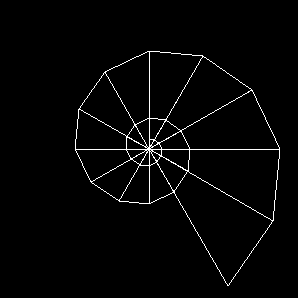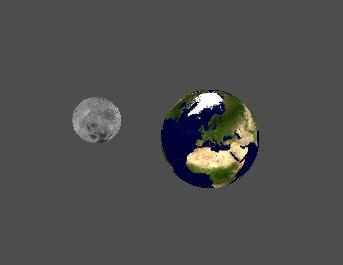programming / C++ / sailing / nerd stuff
glua

## Description

This chapter gives an overview of the tool and what it supports, regarding OpenGL, GLU and GLUT.

### Introduction

This tool is to play with OpenGL, without having to compile every single bit. It is certainly not optimized to maximum speed, nor does it implement the latest and greatest standard.

For beginners and their first steps it may be helpful.

Supported are many of the functions of OpenGL 1.1, GLU (GL utility), and GLUT (GL utility toolkit).

Please Note: this is not an introduction into OpenGL, and is directed to people already knowing how to use a compiler, build tools, etc. Constructive comments are always welcome.

### OpenGL Support

All of the following GLUT function are supported. This list contains the original names given by OpenGL.

The namespace within the script is `gl` and the functions themselfs have no prefix `glut`. Example: using the function `glBegin` within the script is changed to `gl.Begin`.

`glClearIndex`, `glClearColor`, `glClear`, `glIndexMask`, `glColorMask`, `glAlphaFunc`, `glBlendFunc`, `glLogicOp`, `glCullFace`, `glFrontFace`, `glPointSize`, `glLineWidth`, `glLineStipple`, `glPolygonMode`, `glPolygonOffset`, `glEdgeFlag`, `glScissor`, `glClipPlane`, `glGetClipPlane`, `glDrawBuffer`, `glReadBuffer`, `glEnable`, `glDisable`, `glIsEnabled`, `glEnableClientState`, `glDisableClientState`, `glGetBooleanv`, `glGetDoublev`, `glGetFloatv`, `glGetIntegerv`, `glPushAttrib`, `glPopAttrib`, `glPushClientAttrib`, `glPopClientAttrib`, `glRenderMode`, `glGetError`, `glFinish`, `glFlush`, `glHint`, `glClearDepth`, `glDepthFunc`, `glDepthMask`, `glDepthRange`, `glClearAccum`, `glAccum`, `glMatrixMode`, `glOrtho`, `glFrustum`, `glViewport`, `glPushMatrix`, `glPopMatrix`, `glLoadIdentity`, `glLoadMatrixd`, `glLoadMatrixf`, `glMultMatrixd`, `glMultMatrixf`, `glRotated`, `glRotatef`, `glScaled`, `glScalef`, `glTranslated`, `glTranslatef`, `glIsList`, `glDeleteLists`, `glGenLists`, `glNewList`, `glEndList`, `glCallList`, `glCallLists`, `glListBase`, `glBegin`, `glEnd`, `glVertex2d`, `glVertex2f`, `glVertex2i`, `glVertex2s`, `glVertex3d`, `glVertex3f`, `glVertex3i`, `glVertex3s`, `glVertex4d`, `glVertex4f`, `glVertex4i`, `glVertex4s`, `glVertex2dv`, `glVertex2fv`, `glVertex2iv`, `glVertex2sv`, `glVertex3dv`, `glVertex3fv`, `glVertex3iv`, `glVertex3sv`, `glVertex4dv`, `glVertex4fv`, `glVertex4iv`, `glVertex4sv`, `glNormal3b`, `glNormal3d`, `glNormal3f`, `glNormal3i`, `glNormal3s`, `glNormal3bv`, `glNormal3dv`, `glNormal3fv`, `glNormal3iv`, `glNormal3sv`, `glIndexd`, `glIndexf`, `glIndexi`, `glIndexs`, `glIndexub`, `glIndexdv`, `glIndexfv`, `glIndexiv`, `glIndexsv`, `glIndexubv`, `glColor3b`, `glColor3d`, `glColor3f`, `glColor3i`, `glColor3s`, `glColor3ub`, `glColor3ui`, `glColor3us`, `glColor4b`, `glColor4d`, `glColor4f`, `glColor4i`, `glColor4s`, `glColor4ub`, `glColor4ui`, `glColor4us`, `glColor3bv`, `glColor3dv`, `glColor3fv`, `glColor3iv`, `glColor3sv`, `glColor3ubv`, `glColor3uiv`, `glColor3usv`, `glColor4bv`, `glColor4dv`, `glColor4fv`, `glColor4iv`, `glColor4sv`, `glColor4ubv`, `glColor4uiv`, `glColor4usv`, `glTexCoord1d`, `glTexCoord1f`, `glTexCoord1i`, `glTexCoord1s`, `glTexCoord2d`, `glTexCoord2f`, `glTexCoord2i`, `glTexCoord2s`, `glTexCoord3d`, `glTexCoord3f`, `glTexCoord3i`, `glTexCoord3s`, `glTexCoord4d`, `glTexCoord4f`, `glTexCoord4i`, `glTexCoord4s`, `glTexCoord1dv`, `glTexCoord1fv`, `glTexCoord1iv`, `glTexCoord1sv`, `glTexCoord2dv`, `glTexCoord2fv`, `glTexCoord2iv`, `glTexCoord2sv`, `glTexCoord3dv`, `glTexCoord3fv`, `glTexCoord3iv`, `glTexCoord3sv`, `glTexCoord4dv`, `glTexCoord4fv`, `glTexCoord4iv`, `glTexCoord4sv`, `glRasterPos2d`, `glRasterPos2f`, `glRasterPos2i`, `glRasterPos2s`, `glRasterPos3d`, `glRasterPos3f`, `glRasterPos3i`, `glRasterPos3s`, `glRasterPos4d`, `glRasterPos4f`, `glRasterPos4i`, `glRasterPos4s`, `glRasterPos2dv`, `glRasterPos2fv`, `glRasterPos2iv`, `glRasterPos2sv`, `glRasterPos3dv`, `glRasterPos3fv`, `glRasterPos3iv`, `glRasterPos3sv`, `glRasterPos4dv`, `glRasterPos4fv`, `glRasterPos4iv`, `glRasterPos4sv`, `glRectd`, `glRectf`, `glRecti`, `glRects`, `glRectdv`, `glRectfv`, `glRectiv`, `glRectsv`, `glShadeModel`, `glLightf`, `glLighti`, `glLightfv`, `glLightiv`, `glGetLightfv`, `glGetLightiv`, `glLightModelf`, `glLightModeli`, `glLightModelfv`, `glLightModeliv`, `glMaterialf`, `glMateriali`, `glMaterialfv`, `glMaterialiv`, `glGetMaterialfv`, `glGetMaterialiv`, `glColorMaterial`, `glPixelZoom`, `glPixelStoref`, `glPixelStorei`, `glPixelTransferf`, `glPixelTransferi`, `glPixelMapfv`, `glPixelMapuiv`, `glPixelMapusv`, `glDrawPixels`, `glCopyPixels`, `glStencilFunc`, `glStencilMask`, `glStencilOp`, `glClearStencil`, `glTexGend`, `glTexGenf`, `glTexGeni`, `glTexGendv`, `glTexGenfv`, `glTexGeniv`, `glTexParameterf`, `glTexParameteri`, `glTexParameterfv`, `glTexParameteriv`, `glTexImage1D`, `glTexImage2D`, `glFogf`, `glFogi`, `glFogfv`, `glFogiv`, `glInitNames`, `glLoadName`, `glPushName`, `glPopName`, `glGenTextures`, `glDeleteTextures`, `glBindTexture`, `glTexSubImage1D`, `glTexSubImage2D`, `glCopyTexImage1D`, `glCopyTexImage2D`, `glCopyTexSubImage1D`, `glCopyTexSubImage2D`

### GLU Support

All of the following GLUT function are supported. This list contains the original names given by GLU.

The namespace within the script is `glu` and the functions themselfs have no prefix `glut`. Example: using the function `gluLookAt` within the script is changed to `glu.LookAt`.

`gluPerspective`, `gluLookAt`, `gluNewQuadric`, `gluDeleteQuadric`, `gluSphere`, `gluQuadricNormals`, `gluQuadricTexture`

### GLUT Support

All of the following GLUT function are supported. This list contains the original names given by GLUT.

The namespace within the script is `glut` and the functions themselfs have no prefix `glut`. Example: using the function `glutInitWindowSize` within the script is changed to `glut.InitWindowSize`.

`glutRegisterCallback`, `glutInitWindowSize`, `glutInitWindowPosition`, `glutInitDisplayMode`, `glutCreateWindow`, `glutDisplayFunc`, `glutReshapeFunc`, `glutKeyboardFunc`, `glutMouseFunc`, `glutMotionFunc`, `glutIdleFunc`, `glutSpecialFunc`, `glutMainLoop`, `glutSwapBuffers`, `glutPostRedisplay`, `glutReshapeWindow`, `glutPositionWindow`, `glutFullScreen`, `glutWireSphere`, `glutWireCube`, `glutWireCone`, `glutWireTorus`, `glutWireDodecahedron`, `glutWireTeapot`, `glutWireOctahedron`, `glutWireTetrahedron`, `glutWireIcosahedron`, `glutSolidSphere`, `glutSolidCube`, `glutSolidCone`, `glutSolidTorus`, `glutSolidDodecahedron`, `glutSolidTeapot`, `glutSolidOctahedron`, `glutSolidTetrahedron`, `glutSolidIcosahedron`

### Miscellaneous

There are other functions provided to be used within scripts:

• `img.load_bmp`
• `img.free_img`
• `util.timeusec`
• `util.quit_app`

## Usage

Just start the program with the script you like to execute as parameter, example:

``\$ ./glua simple.lua``

## Examples

This chapter shows two examples how to use glua. There is not an example for every supported GL/GLU/GLUT function, but you’ll get the idea.

### Simple

This is a very simple example that shows the bare minimum. A nice helix on black ground is drawn.

File: simple.luaThe display function is very straight forward. Clear buffers, position observer (line 5), then draw a helix with growing radius.

``````display = function()
gl.Clear(gl._COLOR_BUFFER_BIT)
gl.Translatef(0.0, 0.0, 10.0)

local r = 10

gl.Color4f(1.0, 1.0, 1.0, 1.0)
gl.Begin(gl._TRIANGLE_FAN)
gl.Vertex2i(0, 0)
for phi = 0,870,30 do
local rad = phi * math.pi / 180.0
r = r * 1.1
end
gl.End()

gl.Flush()
end``````

The following function handles the resize of the window. GLUT also uses this function to initialize the GL part and is therefore mandatory. In this case we use an orthogonal projection.

``````reshape = function(w, h)
gl.Viewport(0, 0, w, h)
gl.MatrixMode(gl._PROJECTION)
gl.Ortho(-150.0, 150.0, -150.0, 150.0, -150.0, 150.0)
gl.MatrixMode(gl._MODELVIEW)
end``````

The `keyboard` function listens for `q`,`Q`,`ESCAPE` buttons and quits the demo if occurring.

``````keyboard = function(c, x, y)
if c == util.KEY_q or c == util.KEY_Q or c == util.KEY_ESC then
util.quit_app()
end
end``````

The initialization is nothing special. We tell GLUT to create a window with a certain size and position.

Be aware of the non-GLUT function on line 40 (marked bold). This class is necessary to let glua register the callback functions within GLUT. Everything else is just plain GL, GLU and GLUT.

``````    glut.InitWindowSize(300, 300)
glut.InitDisplayMode(glut._RGBA)
glut.InitWindowPosition(0, 0)
glut.CreateWindow('Simple')
glut.RegisterCallback()
glut.DisplayFunc('display')
glut.ReshapeFunc('reshape')
glut.KeyboardFunc('keyboard')
gl.ClearColor(0.0, 0.0, 0.0, 1.0)
gl.PolygonMode(gl._FRONT_AND_BACK, gl._LINE)

glut.MainLoop()``````

Run this script like:

``\$ ./glua simple.lua``

### Earth and Moon

This example shows two spheres (earth and moon) in rotation. The usage of texture mapping and quadrics (GLU) are shown as well as time-based animation.

File: earthmoon.luaThe first lines are initialization of some values:

``````    tex = gl.textures()
accel = 0.5
omega_earth = 504.0
phi_earth = 0.0
img_earth = nil
tex_earth = nil
omega_earth_moon = 18.0
phi_earth_moon = 0.0
omega_moon = 18.0
phi_moon = 0.0
img_moon = nil
tex_moon = nil
timestamp = util.time_usec()``````

The function `display` is used to draw the scene. In this case it is two spheres, each with a texture. One sphere shows the earth, the other the moon. The distance between them and the rotations are not accurate.

First set the observer position and view direction (lines 20-24), then draw the first sphere (lines 27-34), draw the second sphere (lines 37-46) and finally let the buffers swap.

``````display = function()
gl.Clear(gl._COLOR_BUFFER_BIT + gl._DEPTH_BUFFER_BIT)
glu.LookAt(
0.0, -20.0, 20.0,
0.0,   0.0,  0.0,
0.0,   1.0,  0.0
)

-- earth
gl.PushMatrix()
gl.Color4fv( { 1.0, 1.0, 1.0, 1.0 } )
gl.BindTexture(gl._TEXTURE_2D, tex_earth)
gl.Rotatef(phi_earth, 0.0, 0.0, 1.0)
gl.PopMatrix()
end

-- moon
gl.PushMatrix()
gl.Color4fv( { 1.0, 1.0, 1.0, 1.0 } )
gl.Rotatef(phi_earth_moon, 0.0, 0.0, 1.0)
gl.Translatef(10.0, 0.0, 0.0)
gl.BindTexture(gl._TEXTURE_2D, tex_moon)
gl.Rotatef(phi_moon, 1.0, 1.0, 1.0)
gl.PopMatrix()
end

gl.Flush()
glut.SwapBuffers()
end``````

This function sets the viewport and projection options.

``````reshape = function(w, h)
gl.Viewport(0, 0, w, h)
gl.MatrixMode(gl._PROJECTION)
glu.Perspective(60.0, w/h, 0.1, 100.0)
gl.MatrixMode(gl._MODELVIEW)
end``````

The `keyboard` function listens for `q`,`Q`,`ESCAPE` buttons and quits the demo if occurring.

``````keyboard = function(c, x, y)
if c == util.KEY_q or c == util.KEY_Q or c == util.KEY_ESC then
util.quit_app()
end
end``````

Function to increment/decrement angles and keeping them between 0 and 360 degrees.

``````delta_phi = function(phi, delta)
local p = phi + delta
if p > 0.0 then
while p > 360.0 do p = p - 360.0 end
else
while p < 0.0 do p = p + 360.0 end
end
return p
end``````

Whenever GLUT has some time to spare, this function will be called. It calculates the time since the last time the angles were altered and increases the angles for earth, the moon and the moon around the earth accordingly. At last it asks GLUT to redraw the scene.

``````idle = function()
local t = util.time_usec()
local dt = accel * 0.000001 * (timestamp - t)
timestamp = t

phi_earth = delta_phi(phi_earth, -(omega_earth * dt))
phi_moon = delta_phi(phi_moon, -(omega_moon * dt))
phi_earth_moon = delta_phi(phi_earth_moon, -(omega_earth_moon * dt))
glut.PostRedisplay()
end``````

The final part does not reside within a function. It handles the entire GL/GLUT initialization, loads textures and creates the GLU quadrics.

The only lines special to glua are 94, 111 and 119. Those are used to register the GLUT callback functions and get the texutre handle out of the array `tex`. Once you get the IDs, they can be used as normal.

``````    img_earth = img.load_bmp('earth.bmp')

glut.InitWindowSize(600, 400)
glut.InitDisplayMode(glut._RGBA + glut._DOUBLE + glut._DEPTH)
glut.InitWindowPosition(0, 0)
glut.CreateWindow('Earth and Moon')
glut.RegisterCallback()
glut.DisplayFunc('display')
glut.ReshapeFunc('reshape')
glut.KeyboardFunc('keyboard')
glut.IdleFunc('idle')
gl.ClearColor(0.3, 0.3, 0.3, 1.0)
gl.ClearDepth(1.0)
gl.Enable(gl._DEPTH_TEST)
gl.Enable(gl._NORMALIZE)
gl.Enable(gl._TEXTURE_2D)
gl.BlendFunc(gl._ONE, gl._ONE)
gl.CullFace(gl._BACK)
gl.FrontFace(gl._CCW)
gl.PolygonMode(gl._FRONT_AND_BACK, gl._FILL)

gl.GenTextures(2, tex)

tex_earth = gl.tex(tex, 0)
gl.BindTexture(gl._TEXTURE_2D, tex_earth)
gl.TexImage2D(gl._TEXTURE_2D, 0, 4, img.image_width(img_earth),
img.image_height(img_earth), 0, gl._RGBA, gl._UNSIGNED_BYTE,
img.image_data(img_earth))
gl.TexParameteri(gl._TEXTURE_2D, gl._TEXTURE_MIN_FILTER, gl._LINEAR)
gl.TexParameteri(gl._TEXTURE_2D, gl._TEXTURE_MAG_FILTER, gl._LINEAR)

tex_moon = gl.tex(tex, 1)
gl.BindTexture(gl._TEXTURE_2D, tex_moon)
gl.TexImage2D(gl._TEXTURE_2D, 0, 4, img.image_width(img_moon),
img.image_height(img_moon), 0, gl._RGBA, gl._UNSIGNED_BYTE,
img.image_data(img_moon))
gl.TexParameteri(gl._TEXTURE_2D, gl._TEXTURE_MIN_FILTER, gl._LINEAR)
gl.TexParameteri(gl._TEXTURE_2D, gl._TEXTURE_MAG_FILTER, gl._LINEAR)

glut.MainLoop()``````

The following lines will not be executed, but they are here to show how to destroy the quadrics and textures.

``````    glu.DeleteQuadric(quad_moon)
gl.DeleteTextures(2, tex)
img.free_img(img_earth)
img.free_img(img_moon)``````

All source code provided by this page is distributed unter the terms of the GPL2.

This source distribution does not include any OpenGL, GLU or GLUT components. It does contain the complete LUA stuff.

``\$ make``
The `Makefile` tries to determine the platform (one of the supported) and sets all variables accordingly.